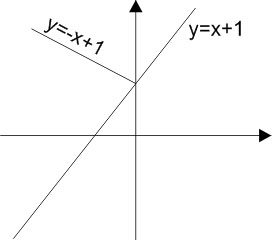# Let be a function defined by Then which of the following is true ? Option 1) is differentiable everywhere Option 2) is not differentiable at Option 3) Option 4) is not differentiable at

As we learnt in

Condition for differentiable -

A function  f(x) is said to be differentiable at    if      both exist and are equal otherwise non differentiable

-So f(x) is differentiable every where.

Option 1)

is differentiable everywhere

Correct

Option 2)

is not differentiable at

Incorrect

Option 3)

Incorrect

Option 4)

is not differentiable at

Incorrect

Exams
Articles
Questions